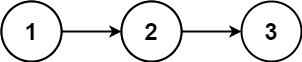# 382. Linked List Random Node#

## 题目 #

Given a singly linked list, return a random node’s value from the linked list. Each node must have the same probability of being chosen.

Implement the `Solution` class:

• `Solution(ListNode head)` Initializes the object with the integer array nums.
• `int getRandom()` Chooses a node randomly from the list and returns its value. All the nodes of the list should be equally likely to be choosen.

Example 1:``````Input
["Solution", "getRandom", "getRandom", "getRandom", "getRandom", "getRandom"]
[[[1, 2, 3]], [], [], [], [], []]
Output
[null, 1, 3, 2, 2, 3]

Explanation
Solution solution = new Solution([1, 2, 3]);
solution.getRandom(); // return 1
solution.getRandom(); // return 3
solution.getRandom(); // return 2
solution.getRandom(); // return 2
solution.getRandom(); // return 3
// getRandom() should return either 1, 2, or 3 randomly. Each element should have equal probability of returning.

``````

Constraints:

• The number of nodes in the linked list will be in the range `[1, 104]`.
• `-10^4 <= Node.val <= 10^4`
• At most `10^4` calls will be made to `getRandom`.

• What if the linked list is extremely large and its length is unknown to you?
• Could you solve this efficiently without using extra space?

## 解题思路 #

• rand.Float64() 可以返回 [0.0,1.0) 之间的随机数。利用这个函数完成我们的随机化取节点的过程。

## 代码 #

``````package leetcode

import (
"math/rand"

"github.com/halfrost/leetcode-go/structures"
)

// ListNode define
type ListNode = structures.ListNode

/**
* type ListNode struct {
*     Val int
*     Next *ListNode
* }
*/
type Solution struct {
}

Note that the head is guaranteed to be not null, so it contains at least one node. */
}

/** Returns a random node's value. */
func (this *Solution) GetRandom() int {
scope, selectPoint, curr := 1, 0, this.head
for curr != nil {
if rand.Float64() < 1.0/float64(scope) {
selectPoint = curr.Val
}
scope += 1
curr = curr.Next
}
return selectPoint
}

/**
* Your Solution object will be instantiated and called as such:Apr 8, 2023Edit this page